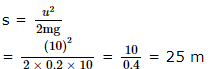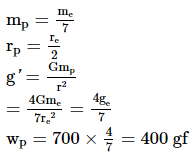Courses

# SRMJEEE Chemistry Mock Test - 3

## 35 Questions MCQ Test SRMJEEE Subject Wise & Full Length Mock Tests | SRMJEEE Chemistry Mock Test - 3

Description
This mock test of SRMJEEE Chemistry Mock Test - 3 for JEE helps you for every JEE entrance exam. This contains 35 Multiple Choice Questions for JEE SRMJEEE Chemistry Mock Test - 3 (mcq) to study with solutions a complete question bank. The solved questions answers in this SRMJEEE Chemistry Mock Test - 3 quiz give you a good mix of easy questions and tough questions. JEE students definitely take this SRMJEEE Chemistry Mock Test - 3 exercise for a better result in the exam. You can find other SRMJEEE Chemistry Mock Test - 3 extra questions, long questions & short questions for JEE on EduRev as well by searching above.
QUESTION: 1

Solution:
QUESTION: 2

Solution:
QUESTION: 3

### Which acid is present in lemon?

Solution:
QUESTION: 4

The specific resistance of a wire is ρ, its volume is 3 m3 and its resistance is 3 ohms, then its length will be

Solution:
QUESTION: 5

The isotope atoms differ in ?

Solution:
QUESTION: 6

A metal plate gets heated, when cathode rays against it, due to

Solution:
QUESTION: 7

What is a mixture of potassium nitrate powdered charcoal and sulphur called?

Solution:
QUESTION: 8

Which is also called Stranger Gas ?

Solution:
QUESTION: 9

A CE amplifier has a voltage gain of 50, an input impedance of 1000 ohm, and an output impedance of 200 ohm. The power gain of the amplifier will be:

Solution:
QUESTION: 10

When two capacitors of capacities 3 μ F and 6 μ F are connected in series and connected to 120V, the potential difference across 3 μ F is

Solution:
QUESTION: 11

On a rough horizontal surface, a body of mass 2 kg is given a velocity of 10 m/s. If the coefficient of friction is 0.2 and g = 10 m/s2, the body will stop after covering a distance of

Solution:QUESTION: 12

The r.m.s. speed of the molecules of a gas in a vessel is 400 ms⁻1. If half of the gas leaks out, at constant temperature, the r.m.s. speed of the remaining molecules will be

Solution:
QUESTION: 13

In a room where the temprature is 30°C, a body cods from 61°C to 59°C in 4 minutes. The time (in min.) taken by the body to cool from 51°C to 49°C will be

Solution:
QUESTION: 14

An ammeter and a voltmeter of resistance R are connected in series to an electric cell of negligible internal resistance. Thier readings are A and V respectively. If another resistance R is connected in parallel with the voltmeter.

Solution:
QUESTION: 15

Which of the following is NOT an illustration of Newton's third law ?

Solution:
QUESTION: 16

What is the weight of a 700 g of body on a planet whose is 1/7 th of that of earth and radius is 1/2 times of earth ?

Solution:QUESTION: 17

The incorrect statement regarding the lines of force of the magnetic field B is

Solution:
QUESTION: 18

A beaker containing a liquid of density (ρ) moves up with an acceleration (a). The pressure due to liquid at a depth (h) below the surface of the liquid is

Solution:
QUESTION: 19

An aeroplane is moving with horizontal velocity u at height h. The velocity of a packet dropped from it on the earth's surface will be (g is acceleration due to gravity)

Solution:
Initially, the pocket has an only horizontal velocity equal to u
When it reaches the ground it has horizontal velocity u and vertical velocity 2gh√
Therefore resultant velocity =√u^2+2gh
Hence A is the correct answer.
QUESTION: 20

A ball is rolled off along the edge of table with velocity 4 m/s. It hits the ground after time 0.4 s. Which one of the following is wrong? (g = 10 m/s2)

Solution:
QUESTION: 21

A boy whose mass is 50 kg stands on a spring balance inside a lift. The lift starts to ascent with an acceleration of 2ms⁻1. The reading of the machine or balance (g = 10 ms⁻2) is

Solution:
QUESTION: 22

If a spring extends by x on loading, then the energy stored by the spring is (if T is tension in the spring and k is spring constant)

Solution:
QUESTION: 23

Two points particles with masses m₁ and m₂ are thrown at angles θ₁ and θ₂ with the horizontal with speed ν₁ and ν₂ respectively. T,H and R represent the total time of flight, the maximum height attained and the horizontal range covered respectively. If ν₁ sin θ₁ = ν₂ sin θ₂, then for both the paricles

Solution:
QUESTION: 24

The total weight of a piece of wood is 6 kg. In the floating state in water its 1/3 part remains inside the water. On this floating solid, what maximum weight is to be put such that the whole of the piece of wood is to be drowned in the ratio?

Solution:
QUESTION: 25

The distance between eye lens and cross wire in Ramsden eye piece which has a field lens of focal length 1.2 cm is .......cm.

Solution:
QUESTION: 26

A stone is tied up to the end of a string and whirled in a vertical circle. The tension in the string is T1 when the stone is at the highest point and T2 when it is at the lowest point. Then

Solution:

QUESTION: 27

Newton per square metre is the unit of

Solution:
QUESTION: 28

The surface tension of soap solution is 25x10⁻3Nm⁻1. The excess pressure inside a soap bubble of diameter 1 cm is

Solution:
QUESTION: 29

A current i passes through a wire of length l, radius of cross-section r and density ρ. The rate of heat generation is

Solution:
QUESTION: 30

For a perfect gas, if α and β are volume and pressure coefficients of expansion.

Solution:
QUESTION: 31

It is difficult to cook rice in an open vessel by boiling it at high altitudes because of

Solution:
QUESTION: 32

Which of the following is not the unit of time?

Solution:
QUESTION: 33

The penetration of light into the region of geometrical shadow is called

Solution:
QUESTION: 34

A source and a listener are moving towards each other with a speed 1/10th of the speed of sound. if the frequency of the note emitted by the source is f, the frequency of the note heard by the listner will be nearly

Solution:
QUESTION: 35

A body of mass 10 kg at rest is acted upon simultaneously by two forces 4 N and 3 N at right angles to each to each other. The kinetic energy of the body at the end of 10 sec is

Solution: